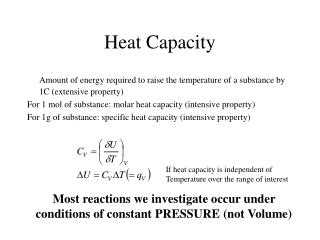DownloadDownload PresentationHeat Capacity

# Heat Capacity

Télécharger la présentation## Heat Capacity

- - - - - - - - - - - - - - - - - - - - - - - - - - - E N D - - - - - - - - - - - - - - - - - - - - - - - - - - -
##### Presentation Transcript

1. Heat Capacity Amount of energy required to raise the temperature of a substance by 1C (extensive property) For 1 mol of substance: molar heat capacity (intensive property) For 1g of substance: specific heat capacity (intensive property) If heat capacity is independent of Temperature over the range of interest Most reactions we investigate occur under conditions of constant PRESSURE (not Volume)

2. Enthalpy Heat of reaction at constant pressure! Use a “coffee-cup” calorimeter to measure it Heat capacity

3. U and H Only differ significantly when gases are involved Standard Enthalpy Changes, Ho H for a process in which the initial and final species are in their standard states. Can be reported for any T. Use 298K unless otherwise indicated Hvapo:1 mole pure liquid vapourises to a gas at 1bar (+40.66 kJmol-1 at 373K for water) Hfuso:1mole pure solid melts to a pure liquid at 1bar (+6.01 kJmol-1 at 273K for ice)

4. Standard Enthalpy Changes CaO(s) + CO2(g) ---> CaCO3(s) rxnHo = -178.3kJmol-1 Thermochemical equations: standard heats of reaction, rxnHo Hess’s Law If a reaction is the sum of two separate reactions then the enthalpy change during that reaction is also the sum of the enthalpy changes in the component reactions.

5. Hess’s Law rxnHo = Hoproducts - Horeactants

6. Standard Heats of Formation If one mole of the compound is formed under standard conditions from its elements in their standard state then the resulting enthalpy change is said to be the standard molar enthalpy (Heat) of formation, fHo where the subscript indicates this. By definition the enthalpies of formation of the elements in their standard states are zero. H2 (g) + 1/2O2 (g) ----> H2O (l) fHo = -285.8kJmol-1 2C (s) + 3H2(g) + 1/2O2(g) -----> C2H5OH (l) fHo = -277.7kJmol-1

7. Hess’s Law Hess‘s Law is particularly useful for calculating fHo which would not be easy to measure experimentally. fHo for CO cannot be measured as CO2 is also formed when graphite is burned C(s) + 1/2O2 ----> CO fHo = x CO + 1/2O2 ----> CO2rxnHo = -283 kJmol-1 _______________________________________ C(s) + O2 ----> CO2fHo = -393.5 kJmol-1 From looking at these equations it is fairly obvious that the sum of the first two enthalpies is equal to the third by Hess‘s Law. i.e. x - 283 = -393.5 or x = -110.5 kJmol-1.

8. Enthalpy Changes and Bond Energies Energy is absorbed when bonds break. The energy required to break the bonds is absorbed from the surroundings. If there was some way to figure out how much energy a single bond absorbed when broken, the enthalpy of reaction could be estimated by subtracting the bond energies for bonds formed from the total bond energies for bonds broken. O2(g) 2O(g) H°=490.4 kJ H2(g) 2H(g) H° =431.2 kJ H2O(g)2H(g) + O(g) H°=915.6 kJ We can estimate the bond enthalpies of O=O, H-H, and O-H as 490.4 kJ/mol, 431.2 kJ/mol, and 457.7 kJ/mol, respectively. 2H2(g) + O2(g)  2H2O(g) H°= ?

9. 2H2(g) + O2(g)  2H2O(g) moles of bonds formed moles of bonds broken Energy absorbed Energy released 2 H-H @ 431.2 kJ each 862.4kJ 4 O-H @ 457.7 kJ each 1830.9kJ 1 O=O @ 490.4 kJ each 490.4kJ _____________________________________________ 1352.7kJ 1830.9kJ H°= 1352.7 - 1830.9 kJ = -478.2 kJ. (Remember that the minus sign means "energy released", so you add the bond energies for broken bonds and subtract energies for bonds formed to get the total energy.) A calculation based on enthalpies of formation gave H° = -483.7 kJ Bonds in a molecule influence each other, which means that bond energies aren't really additive. An O-H bond in a water molecule has a slightly different energy than an O-H bond in H2O2, because it's in a slightly different environment. Reaction enthalpies calculated from bond energies are very rough approximations!

10. Thermodynamics and Spontaneity Spontaneous change: the direction of change that does not require work (outside assistance) to bring it about Spontaneous chemical reactions often are exothermic: Are all exothermic reactions spontaneous (Bertholet C19)? Ice melting, water vapourisation, NaI dissolving in water are all spontaneous processes, yet are ENDOthermic. Accompanied by an increase in the “randomness” of the distribution of the particles. An increase in ENTROPY (S) 2nd LAW: Spontaneous changes in the universe are accompanied by an increase in disorder of the universe (cannot win, can only break even) Ssystem + Ssurroundings > 0

11. 3rd LAW The entropy of a perfectly ordered crystalline substance is zero at 0 K (cannot break even) Sometimes enthalpy and entropy work together to favour a spontaneous change. What is their relative importance when they don’t? Use the Gibb’s Free Energy (G) devised by J.W. Gibbs. G is the maximum energy in a change that is free to do work G = H - T.S, therefore, for a change at a constant T G = H - T. S Spontaneous change in a system is accompanied by a negative free energy change

12. Gibb’s Free Energy G = H - T. S At equilibrium, G is zero and Go = -RTlnK